Next: Method of Conjugate Gradients Up: Optimization Previous: Optimization

## Method of Steepest DescentFigure 4.1: The method of Steepest Descent approaches the minimum in a zig-zag manner, where the new search direction is orthogonal to the previous.

The method of Steepest Descent is the simplest of the gradient methods. The choice of direction is where f decreases most quickly, which is in the direction opposite to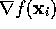. The search starts at an arbitrary point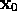and then slide down the gradient, until we are close enough to the solution. In other words, the iterative procedure iswhere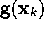is the gradient at one given point. Now, the question is, how big should the step taken in that direction be; i.e. what is the value of? Obviously, we want to move to the point where the function f takes on a minimum value, which is where the directional derivative is zero. The directional derivative is given bySetting this expression to zero, we see thatshould be chosen so that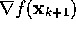andare orthogonal. The next step is then taken in the direction of the negative gradient at this new point and we get a zig-zag pattern as illustrated in Figure (4.1). This iteration continues until the extremum has been determined within a chosen accuracy.

What we have here is actually a minimization problem along a line, where the line is given by (4.7) for different values of. This is usually solved by doing a linear search (also referred to as a line search); i.e. searching for a minimum point along a line. A description of some of these methods is given by Press,Walsh,Shew. Hence, the search for a minimum ofis reduced to a sequence of linear searches. This implementation of the Steepest Descent method are often referred to as the optimal gradient method.

Alternatively, one can start out with a chosen value for, which, if necessary, will be modified during the iterations, making sure that the function decreases at each iteration. This is of course a lot simpler, and often works better, in cases where the calculation using a linear search is laborious. It will take many more iterations to reach the minimum, but each iteration will take much less time than by using a linear search.

The resultant iterative algorithm with a linear search is given in Algorithm 4.1.Figure 4.2: The convergence of the method of Steepest Descent. The step size gets smaller and smaller, crossing and recrossing the valley (shown as contour lines), as it approaches the minimum.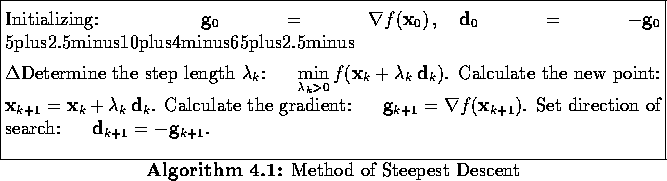As seen, the method of Steepest Descent is simple, easy to apply, and each iteration is fast. It also very stable; if the minimum points exist, the method is guaranteed to locate them after at least an infinite number of iterations. But, even with all these positive characteristics, the method has one very important drawback; it generally has slow convergence. For badly scaled systems; i.e. if the eigenvalues of the Hessian matrixat the solution point are different by several orders of magnitude, the method could end up spending an infinite number of iterations before locating a minimum point. It starts out with a reasonable convergence, but the progress gets slower and slower as the minimum is approached Shew. This is illustrated in Figure 4.2, in the case of a quadratic function with a long, narrow valley. The method may converge fast for such badly scaled systems, but is then very much dependent on a good choice of starting point. In other words, the Steepest Descent method can be used where one has an indication of where the minimum is, but is generally considered to be a poor choice for any optimization problem. It is mostly only used in conjunction with other optimizing methods.Next: Method of Conjugate Gradients Up: Optimization Previous: Optimization

Trond Hjorteland
Mon Jul 5 02:59:28 MET DST 1999